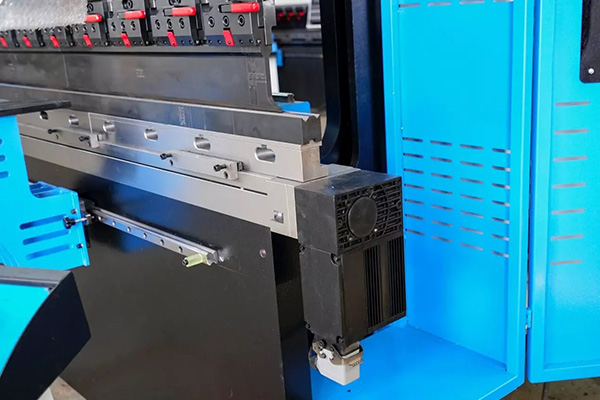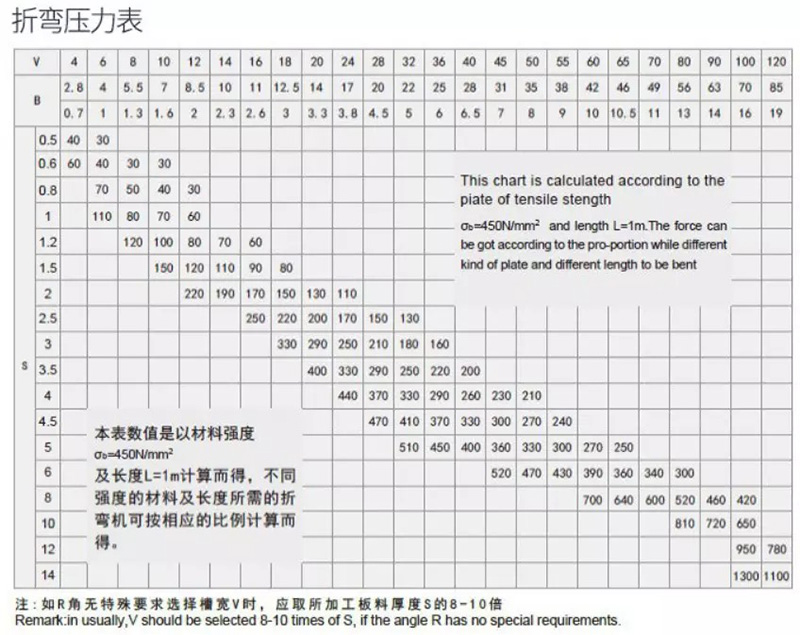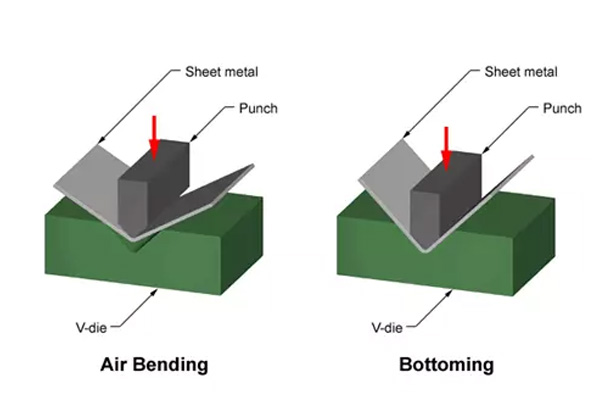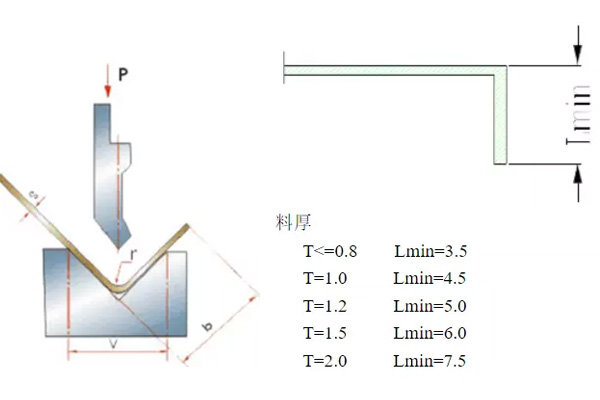# How to Choose the Tonnage of Hydraulic Press Brake Machine

Home / Blog / How to Choose the Tonnage of Hydraulic Press Brake Machine

The tonnage refers to the bending pressure during bending.

Bending is carried out on a machine called a press brake machine, which has a manual and automatic mode. For different plate material and plate thicknesses, there is a range of models from 30T to 2200T available. The bending angle is determined by the depth at which the sheet metal is pressed into the lower die. This depth is precisely controlled to achieve the desired bending. The hydraulic press brake machine is usually equipped with a set of standard molds. Special workpieces need to be customized with special molds. The selection of die material depends on the production quantity, sheet metal material, and bending degree.The first problem of choosing a press brake machine you encounter is how to calculate the bending pressure, which determines how much tonnage you need to buy a sheet metal press brake. Usually, when people calculate hydraulic sheet bending machine tonnage, they can follow the following bending machine tonnage chart.The value in the chart is the bending pressure when the length of the sheet is one meter:

For Example S=4mm L=1000mm V=32mm, Check the table P=330KN. This chart is calculated according to the plate of tensile strength and length L=1m. The force can be got according to the proportion while different kinds of plat and length to be bend. When bending other materials, the bending pressure is the product of the data in the table and the following coefficient.

Bronze (soft): 0.5; Stainless steel: 1.5; aluminum (soft): 0.5; chrome-molybdenum steel: 2.The force required for bending a thin metal plate is calculated by the V-bending method, that is, the thin plate is pressed into the V-shaped die with a V-shaped punch. The bending force can be calculated by sheet thickness, die opening, bending length, and ultimate tensile strength of the material. The die ratio can be entered to calculate the die opening, usually 6 to 12 times of the sheet thickness. Generally, when the thickness is 0-3mm, we use 6 times the sheet thickness. When the thickness is 3-10mm, we use 8 times the sheet thickness. When the thickness is more than 10mm, we use 12 times of the sheet thickness. Then you can use the calculated tonnage to select a suitable sheet metal bending machine.

Approximate calculation formula of bending pressure:P: Bending force (KN)

S: The thickness of the plate (mm)

L: The width of the plate (m)

V: V-width of the bottom die (mm) V is 6-10 times of the thickness of the plate.

Above all, when you calculate the press brake tonnage, you will have two ways: one is to check the press brake tonnage chart and the other is to use the formula.

For example, your plate is S=3mm L=3m, so how much ton you need?

First, we check the press brake tonnage chart, when S=3mm L=1m V=24mm P=250KN.

So, if L=3m, total tonnage is 250KNx3m=750KN=75Ton.

Then we try the Formula, =73Ton. The result is similar to the value we get from the chart. If the plate is stainless steel, the total tonnage is 75Ton x2=150Ton.

Assuming that the thickest material is 1/4 inch, 10 feet of free bending requires 165 tons, and bottomed die bending (corrected bending) requires at least 600 tons. If most parts are 5 feet or shorter, the tonnage is almost halved, which greatly reduces the cost of purchase. The length of the part is very important to determine the specifications of the new press brake machine.

Zhongrui is the China top 10 press brake manufacturers, providing professional press brake bending machine knowledge and high-quality press brake machine for sale. If you have any needs, contact us now!